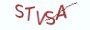# 1.5.2 算法实现

```# __author__ = 薯条老师
import random

# 通过列表推导式来生成一个包含10个随机整数的列表
numbers = [random.randint(0,  100) for _ in range(10)]

# min_表示最小值，min_k2表示次小值
min_, min_k2 = numbers, numbers

for number in numbers[1:]:
if nummber < min_:
# 如果遍历出来的值比min_要小，那么次小值一定是上次的最小值
min_k2 = min_
min_ = number
elif min_ < number < min_k2:
# 如果遍历出来的比min_要大，但比次小值小，那么次小值就为当前遍历出来的值
min_k2 = _
elif min_ == min_k2:
# 如果min_与min_k2刚好为最小值，且相等，就将次小值更新为当前值
min_k2 = number
else:
print("次小值:{}".format(min_k2))```

# 1.5.3 最具实力的小班培训

`(1) Python后端工程师高薪就业班，月薪11K-18K，免费领取课程大纲(2) Python爬虫工程师高薪就业班，年薪十五万，免费领取课程大纲(3) Java后端开发工程师高薪就业班，月薪11K-20K, 免费领取课程大纲(4) Python大数据分析，量化投资就业班，月薪12K-25K,免费领取课程大纲`## 注册账号